## 4. 1. 5 Linear Example - Poisson Equation

This example shows the application of the Poisson equation in a thermodynamic simulation. The equation system consists of four points from which two are boundary points with homogeneous Dirichlet boundary conditions. Furthermore a constant right hand source term is given which equals unity. The four points are given on a straight line in equal distances according to Figure 4.1. The governing equation yields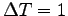for the interior points and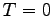for the boundary points.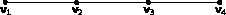In the equation system two unknown variables are defined, namely temperature values for the two inner vertices. The discretization formula resulting from finite differences for the vertices yields(4.15)

The coupling terms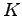yield, if the arguments are identical, unity otherwise. Setting the temperature identically zero at the boundary points leads to the following simplification of the equations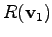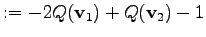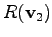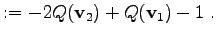(4.16)

The expression for the solution quantity is now replaced by a linearized expression of the following form: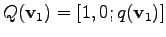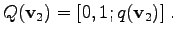(4.17)

Before the linear equation system is solved, the quantity is set to an arbitrary value, for instance zero. For the equations (4.16) one obtains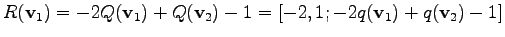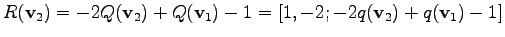(4.18)

It can be seen that the two linear expressionsandcontain the information of the system matrix. The assembly of the matrix from the linear expressions is straight forward, once the linear expressions are obtained.

The solution vector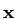contains the single solution elements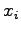. These elements are applied to the quantities according to (4.12). This means, that the solutionis added to the respective solution quantity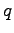. If the initial value of the quantity is zero, the solution quantityhas the same value of the solution of the initially given problem. Otherwise, the solution contains an update vector which has to be added in order to obtain the correct solution. This is equivalent to the Newton method, however, since the equation is linear, the solution is obtained in a single step.

Michael 2008-01-16